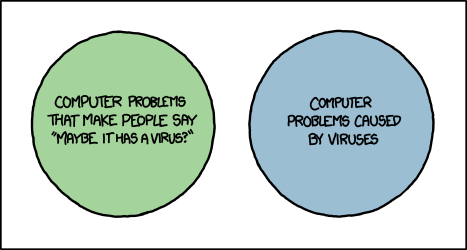This LMS has now been replaced by Arotahi, this site will be used as an archive. You will still be able to login to view but not add content.

# Statistics

• Posted on: 3 June 2021
Block:
Term:
Year:
LA Code:
Code:
Room:
Learning Area:
Levels:
8

We will work on Probability Concepts.This adds several more tools for calculating more complex probability probabilities than you have so far. It leads on from Level 2 Probability, overlapping quite a bit but asking more complex problems and asks you to relate your answers much more to the real life context.
You should have at least a scientific calculator. You could do this course if you haven't done Level 2 maths but you really must have done Level 1 Algebra, Graphs and Chance and Data and be prepared for a lot of extra homework to be able to jump a level.

The exam is external and worth 4 credits.

Achievement
You need to apply probability concepts in solving problems.
This could involve:

• selecting and using methods
• demonstrating knowledge of concepts and terms
• communicating using appropriate representations
• Problems are situations that provide opportunities to apply knowledge or understanding of statistical concepts and methods. Situations will be set in real-life statistical contexts.
You need to be familiar with methods related to:
true probability versus model estimates versus experimental estimates (probabilities to be calculated from formulae, a probability distribution table or graph, tables of counts or proportions, simulation results)

• randomness
• independence
• mutually exclusive events
• conditional probabilities
• probability distribution tables and graphs (probabilities to be calculated from formulae, a probability distribution table or graph, tables of counts or proportions, simulation results)
• two way tables
• probability trees
• Venn diagrams.
• Achievement with Merit
Make sure that you can meet the criteria for achievement
You need to apply probability concepts, using relational thinking, in solving problems.
This could involve one or more of:

• selecting and carrying out a logical sequence of steps
• connecting different concepts or representations
• demonstrating understanding of concepts and also relating findings to a context, or communicating thinking using appropriate statements
• Achievement with Excellence
Make sure that you can meet the criteria for merit
You need to apply probability concepts, using abstract thinking, in solving problems.
this could involve one or more of:

• devising a strategy to investigate or solve a problem
• identifying relevant concepts in context
• developing a chain of logical reasoning
• making a statistical generalisation and also, where appropriate, using contextual knowledge to reflect on the answer.
• Assessment Opportunities: